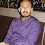# Programming Videos

## Search

### C Program to Print Even Numbers between 1 to 100 using For and While Loop

• Write a C program to print even numbers between 1 to 100 using for and while loop.
• Write a C program to print even numbers between 1 to N.

In this tutorial, we are going to write a c program which prints even numbers between 1 to 100. In another example, we write a c code which prints even numbers between 1 to N (N is an input number by a user).

What is Even Numbers?

An even numbers are those numbers which is exactly divided by 2.

For example - 2, 4 , 6 etc. are even numbers.

C++ program to print even numbers between 1 to 100

Program to print odd and even numbers of an array

Program to check whether number is even or odd

## C Program to Print Even Numbers from 1 to N

```#include <stdio.h>

int main(void) {

int n;

printf ("Enter a number \n");
scanf ("%d", &n);

printf ("Even numbers from 1 to %d is : ",n);

for (int i = 1; i <= n; i++) {

if ( i % 2 == 0) {

printf (" %d ", i);
}

}

return 0;
}

```

## C Program to Print Even Numbers between 1 to 100 using While Loop

```#include <stdio.h>

int main() {

int i=1;

/* Run a loop from 1 to 100 */
while( i <= 100) {

/* If number is divisible by 2
then it's an even number
*/

if(i % 2 == 0){

printf(" %d ", i);
}

/* Increment i. */

i++;
}

return 0;
}
```

Output -

```2  4  6  8  10  12  14  16  18  20  22  24  26  28  30  32  34  36  38  40
42  44  46  48  50  52  54  56  58  60  62  64  66  68  70  72  74  76  78
80  82  84  86  88  90  92  94  96  98  100
```

## C Program to Print Even Numbers between 1 to 100 using For Loop

```#include <stdio.h>

int main() {

/* Run a loop from 1 to 100 */
for(int i = 1; i <= 100; i++) {

/* If number is divisible by 2
then it's an even number
*/
if(i % 2 == 0){

printf(" %d ", i);
}
}

return 0;
}
```

1.Interesting information

2.please describe to show the even and odd number by if else condition in c++

3.Is there any other logic for solving the same program as it is most common logic shown in every websites..��

4.5.6.1.7.